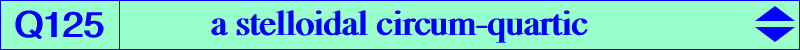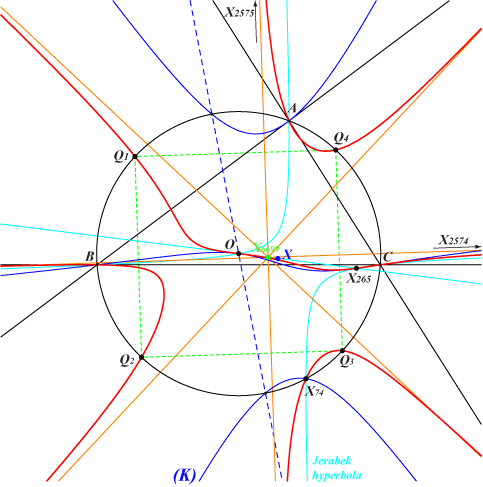too complicated to be written here. Click on the link to download a text file.X(3), X(74), X(265), X(2574), X(2575) anti-points, see Table 77Q125 has four real asymptotes concurring at X(6699), the centroid of {A, B, C, X(74)}, the midpoint of X(3)X(125), also the homothetic of X(265) under h(X3, 1/4). Two are parallel to those of the Jerabek hyperbola and the remaining two are their bisectors. Q125 meets the circumcircle (O) at A, B, C, X(74) and the vertices of a square whose sidelines are also parallel to the asymptotes of the Jerabek hyperbola. The third polar of X(265) is a stelloidal circum-cubic (K) passing through X(3), X(74) hence the tangents at A, B, C, X(3), X(74) concur at X(265). Note that X(3) is a point of inflexion. The radial center X of (K) is the homothetic of X(265) under h(X3, 1/3). (K) meets (O) again on the line X(3)X(924). *** Q125 belongs to a net of stelloidal circum-quartics which contains that decomposed into the McCay cubic K003 and the line at infinity, and also Q085. This net can be generated by three independent nodal stelloidal circum-quartics Q(A), Q(B), Q(C) with nodes A, B, C respectively and passing through X(3). The equation of Q(A) is : 2 y z (c^2 y+b^2 z) (c^2 SC y-b^2 SB z)+x(b^4 z^2 (c^2 (y-x)-(a^2-b^2) z)+c^4 y^2 (b^2(x-z)-(c^2-a^2)y)) = 0, the others are obtained cyclically. Any quartic is then written under the form : Q(P = p:q:r) = p Q(A) + q Q(B) + r Q(C), where P is any point in the plane. Q(X3) corresponds to the McCay case. Q(X23) is Q085 and Q(X74) is Q125. See also Q126, Q127, Q128, Q129, Q130, Q131, Q132, Q133, Q134. The isogonal transforms of Q(A), Q(B), Q(C) are also nodal stelloidal circum-quartics with same nodes. They similarly generate another net of stelloidal quartics passing through X(4). With P = X(2), we obtain the same McCay case. *** Locus property of Q(P) Let M be a variable point, iM its inverse in the circumcircle (O), N the isogonal conjugate of M with respect to the circumcevian triangle of M. The points P, iM, N are collinear if and only if M lies on Q(P) together with (O). *** Properties of Q(P), P ≠ X(3) • The line X(3)P meets the circumcircle (O) at two points P1, P2. The four infinite points of Q(P) are those of the two rectangular circum-hyperbolas passing through P1, P2. It follows that, for any P lying on a fixed line L passing through X(3), the quartics Q(P) have the same points at infinity and form a pencil. • Q(P) always contains A, B, C, X(3) and the inverse iP of P in (O). • The four asymptotes concur at the radial center X of Q(P) which is the centroid of {A, B, C, iP}. X generally does not lie on Q(P) unless P lies on a complicated septic passing through X(3) (triple point), X(23) (see Q085), X(2071) (see Q131), the inverse of X(3146) in (O). See below for a list of pairs {P,X}. • Q(P) passes through P if and only if P lies on K003 or on (O). • Q(P) passes through the isogonal conjugate P* of P if and only if P lies on K003 or on K024. • When P lies on (O), hence iP = P, X lies on the circle C(X140, R/4) and on the tangent at X(3) to Q(P). Q(P) meets (O) again at four points Q1, Q2, Q3, Q4 which are the vertices of a square. The sidelines (resp. diagonals) of this square are parallel to the asymptotes of the rectangular hyperbola passing through P (resp. the antipode of P). • When P lies on the line at infinity, Q(P) meets (O) again at five points Q1, Q2, Q3, Q4, Q5 which are the vertices of a regular pentagon. The radial center is X(140). See Q132, Q133, Q134. The tangent at O to Q(P) is the line OP meeting Q(P) again at two points M1, M2 which are isogonal conjugates hence on the McCay cubic K003. See Q132 for other related loci. • The tangents at A, B, C to Q(P) concur (at Q) if and only if P lies on K039 and then Q lies on K025. If the line OP meets the Stammler hyperbola again at N then Q is the anticomplement of the inverse of N in (O). *** Peter Moses has computed the quartics Q(P) passing through at least four ETC centers (2017-06-24). A few other special cases are also listed. • the yellow cells are those with P on (O). Q(P) contains P and meets (O) at the vertices of a square. • the green cells are those with P at infinity. Q(P) meets (O) at the vertices of a regular pentagon. • the red, blue, green P's lie on the lines passing through X(3) and X(74), X(76), X(8) respectively in which case Q(P) meets the line at infinity at {2574, 2575}, {3413, 3414}, {3307, 3308} respectively. These are the infinite points of the Jerabek, Kiepert, Feuerbach hyperbolas respectively.P = X(i) for i = quartic X(i) on the quartic for i = radial center X 20 Q130 3, 4, 2071 10257 23 Q085 2, 3, 4 2 30 Q132 3, 4 140 74 Q125 3, 74, 265, 2574, 2575 6699 76 3, 3413, 3414, 5152 ? 98 3, 98, 3413, 3414 6036 99 3, 99, 3413, 3414 620 100 3, 100, 3307, 3308 3035 104 3, 104, 3307, 3308 6713 110 3, 110, 2574, 2575 5972 183 2, 3, 3413, 3414, 5939 ? 186 Q129 3, 4 5 399 3, 1511, 2574, 2575 ? 476 Q126 3, 30, 476, 523, 1113, 1114 22104 477 3, 30, 477, 523 ? 517 Q134 1, 3 140 805 Q127 3, 511, 512, 805, 1379, 1380 22103 901 Q128 3, 513, 517, 901, 1381, 1382 22102 927 3, 514, 516, 927 ? 952 Q133 3, 3307, 3308 140 953 3, 513, 517, 953 ? 1145 3, 2932, 3307, 3308 ? 1309 3, 515, 522, 1309 ? 1511 3, 399, 2574, 2575 10272 2071 Q131 3, 4, 20 3 2453 2, 3, 5000, 5001 ? 2698 3, 511, 512, 2698 ? 2724 3, 514, 516, 2724 ? 2734 3, 515, 522, 2734 ? 2867 3, 525, 1503, 2867 ? 2932 3, 1145, 3307, 3308 ? 4996 3, 3307, 3308, 6224 214 5152 3, 76, 3413, 3414 3934 5939 3, 183, 3413, 3414 ? 5976 3, 3413, 3414, 5989 ? 5980 3, 3413, 3414, 5981 ? 5981 3, 3413, 3414, 5980 ? 5989 3, 3413, 3414, 5976 ? 6078 3, 518, 3309, 6078 ? 6079 3, 519, 3667, 6079 ? 6080 3, 520, 6000, 6080 ? 6081 3, 521, 6001, 6081 ? 6082 3, 524, 1499, 6082 ? 6083 3, 758, 6003, 6083 ? 6093 3, 524, 1499, 6093 ? 6224 3, 3307, 3308, 4996 ? 9149 3, 3413, 3414, 9828 ? 9828 3, 3413, 3414, 9149 ? 10620 3, 2574, 2575, 12041 ? 12041 3, 2574, 2575, 10620 ? 12042 3, 3413, 3414, 12188 ? 12188 3, 3413, 3414, 12042 ? 12358 3, 2574, 2575, 12412 ? 12412 3, 2574, 2575, 12358 ?A list of pairs {P,X} by Peter Moses (2017-06-25) {1,6681}, {2,468}, {15,6672}, {16,6671}, {20,10257}, {22,5159}, {23,2}, {36,1125}, {54,10615}, {74,6699}, {98,6036}, {99,620}, {100,3035}, {101,6710}, {102,6711}, {103,6712}, {104,6713}, {105,6714}, {106,6715}, {107,6716}, {108,6717}, {109,6718}, {110,5972}, {111,6719}, {112,6720}, {140,10096}, {186,5}, {187,3589}, {352,10160}, {468,6677}, {858,6676}, {930,13372}, {1155,6690}, {1157,6689}, {1319,6691}, {1324,3634}, {1325,6675}, {1326,6693}, {1511,10272}, {1691,6680}, {2070,3628}, {2071,3}, {2072,10020}, {2073,6678}, {2076,6683}, {2077,6684}, {2078,6692}, {2079,6722}, {3153,7542}, {3455,6698}, {4996,214}, {5152,3934}, {5161,6679}, {5162,10007}, {5172,3812}, {5189,7499}, {5866,141}, {5867,6703}, {5937,6707}, {5940,6}, {5971,10163}, {6104,6669}, {6105,6670}, {6150,8254}, {6636,858}, {7464,549}, {7480,402}, {7488,2072}, {7496,7426}, {7575,547}, {9153,11176}, {10121,10120}, {10257,13383}, {10260,6702}, {10298,10297}, {10989,7495}, {11589,6696}, {11597,11701}, {11643,5461}, {12095,9820}, {13558,6723}.